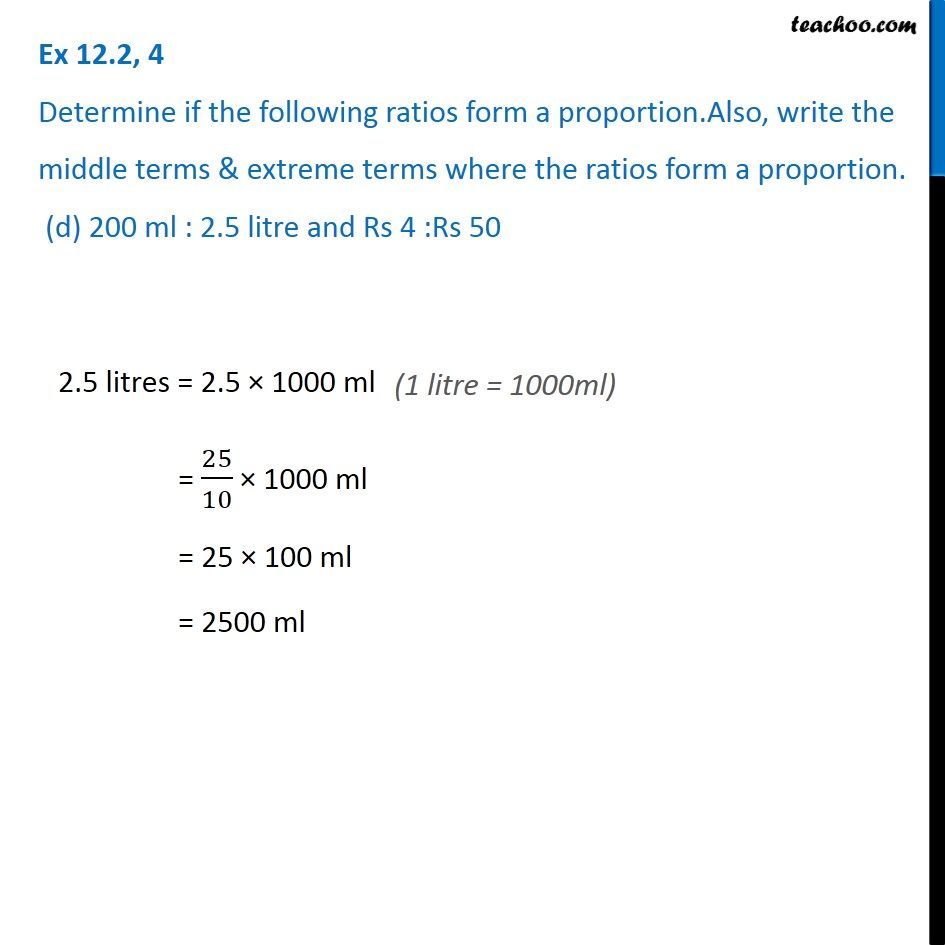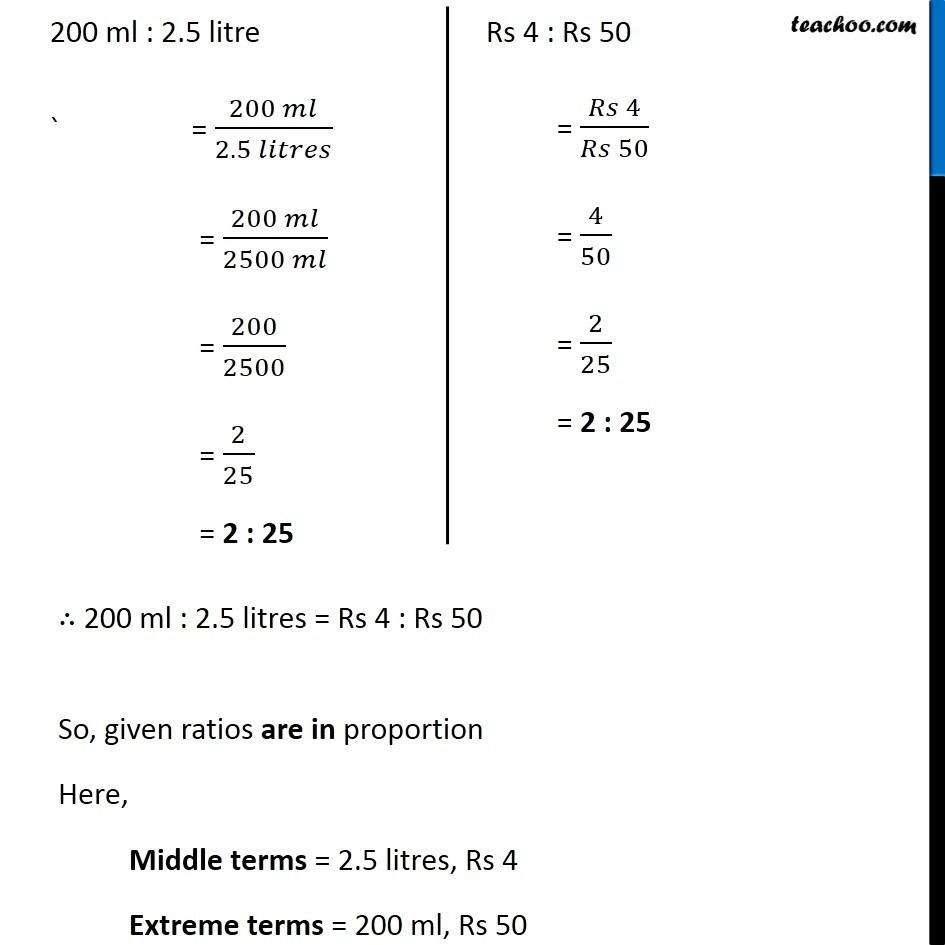Ex 12.2

Chapter 12 Class 6 Ratio And Proportion
Serial order wiseGet live Maths 1-on-1 Classs - Class 6 to 12

### Transcript

Ex 12.2, 4 Determine if the following ratios form a proportion.Also, write the middle terms & extreme terms where the ratios form a proportion. (d) 200 ml : 2.5 litre and Rs 4 :Rs 50 2.5 litres = 2.5 × 1000 ml = 25/10 × 1000 ml = 25 × 100 ml = 2500 ml 200 ml : 2.5 litre ` = (200 𝑚𝑙)/(2.5 𝑙𝑖𝑡𝑟𝑒𝑠) = (200 𝑚𝑙)/(2500 𝑚𝑙) = 200/2500 = 2/25 = 2 : 25 Rs 4 : Rs 50 = (𝑅𝑠 4)/(𝑅𝑠 50) = 4/50 = 2/25 = 2 : 25 ∴ 200 ml : 2.5 litres = Rs 4 : Rs 50 So, given ratios are in proportion Here, Middle terms = 2.5 litres, Rs 4 Extreme terms = 200 ml, Rs 50